Contact Information

William John Elliott S.
56-2-2042863
56-9-85520114
william.john.elliott (contact on Skype)
http://www.wjetech.cl/

wjeconsultant@gmail.com
wje@wjetech.cl

Simple description of proposal (Fluid Space Drive)

Introduction

This document is intended to explain how (and why) a Fluid Space Drive works by presenting the propulsion cycle in 8 simple images.

I understand that you are tempted to reject the proposal “out of hand” because you KNOW it is impossible to accelerate a closed system without expelling mass (very basic physics).

And yet I present a simple method of propulsion that permits spacecraft in a micro-gravity environment to accelerate for extended periods of time in order to obtain a very high velocity without expelling mass. (What has been called a propellantless space drive.) Please read to the end before judging.

Basic elements of a Fluid Space Drive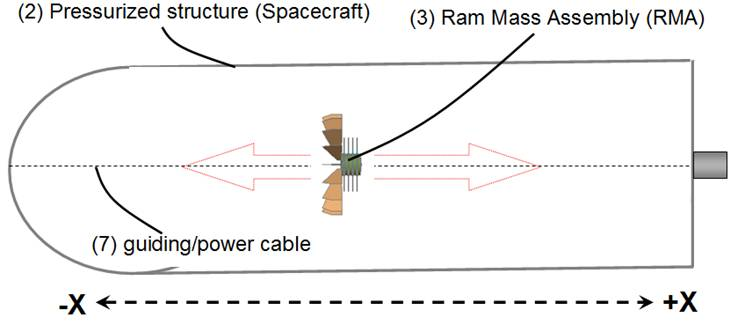Fig 1

Fig 1 shows a pressurized structure (2) or spacecraft in a micro-gravity environment, inside the spacecraft is a 100k mass we call Ram Mass Assembly or RMA.

The RMA has freedom to move across the length (not breadth) of the spacecraft.

For simplicity’s sake we will assume the spacecraft also has a 100k mass. (RMA’s mass = Spacecraft’s mass)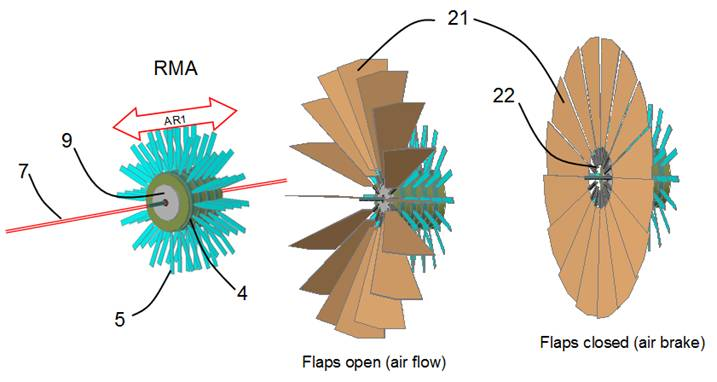Fig 2 RMA

Illustrated (Fig 2) are the main elements of the RMA, electric motor 9, counter rotating rings 4 with rotors/propellers 5, a guiding cable 7 provides power to the motor supplied by a AIP (air independent power unit), also illustrated are a series of flaps 21 attached to servo motors 22, when the flaps are closed they function as an air brake slowing the RMA’s relative velocity inside the pressurized spacecraft.

Propulsion Cycle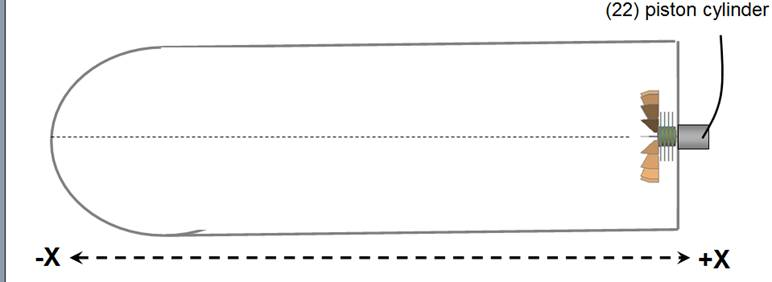Fig 3

Illustrated in Fig 3 is the RMA’s initial position (before starting propulsion cycle) at the forward (+X) end of the pressurized structure, notice there is a piston cylinder 22 in contact with the RMS.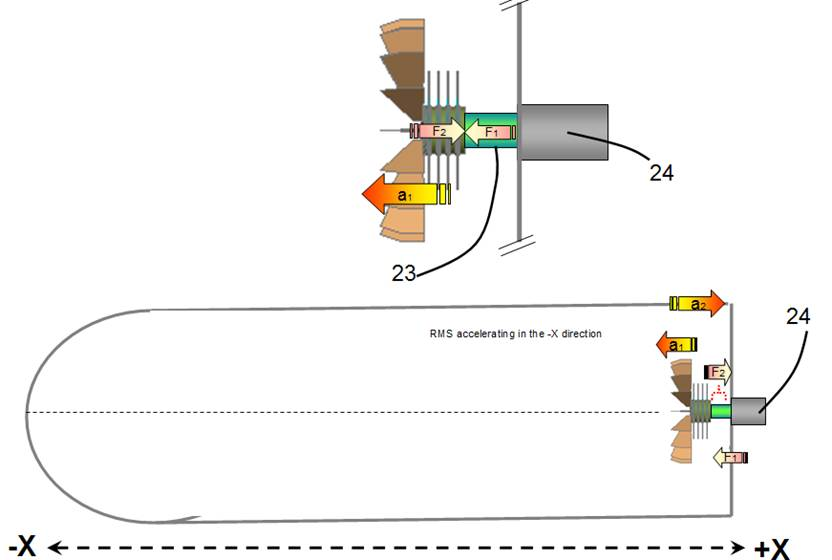Fig 4

Cycle 1

The ramming piston 23 is activated (with a 200n force) giving the RMA a push (F1) in the –X direction (Fig 4) accelerating it in the –X direction (a1) with a velocity of 1 m/s, the opposite force (F2) gives the pressurized structure an acceleration in the +X direction (a2).

Therefore the RMA will have a 1 m/s velocity in the –X direction (V1) and the spacecraft will have a 1 m/s velocity in the +X direction (V2)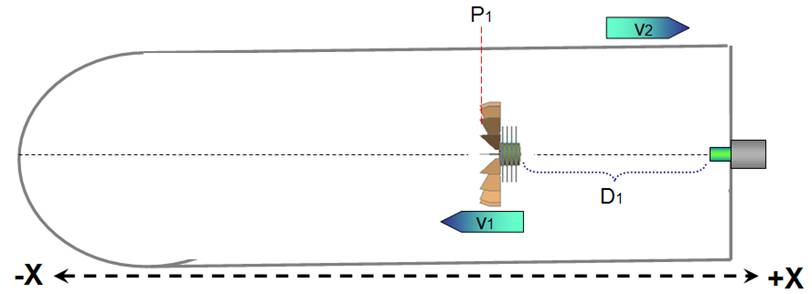Fig 5

Cycle 2

The RMA advances in the –X direction with a constant velocity V1 of 1m/s (RMA’s rotors 5 are off) for a distance (D1) until it reaches a pre programmed position (P1).

(There is no change in velocities V1 and V2 in this cycle)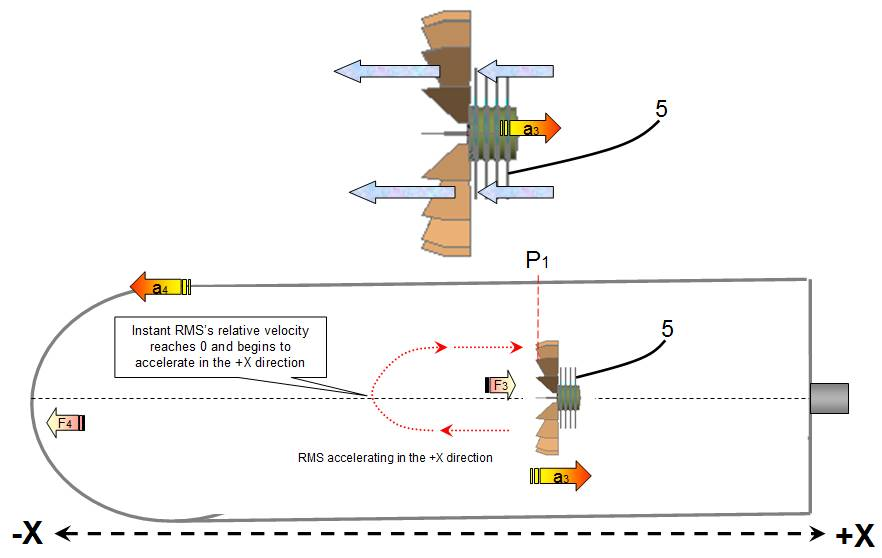Fig 6

Cycle 3

The instant The RMA reaches position P1 (fig 6) its rotor’s 5 are activated blowing air in the –X direction, this creates a force (F3) that accelerate (a3) the RMA in the +X direction

The RMA (that was traveling at constant velocity 1m/s in the –X direction) at first slows (relative to the spacecraft’s velocity) until it reaches a relative velocity of 0 when it begins to gain speed in the +X direction till a pre-programmed velocity (1m/s) is obtained then the rotors stop.

During the time interval the rotors/propellers are on, the flow of air molecules created exert a force F4 against the spacecraft’s –X inner wall accelerating (a4) its velocity in the –X direction, it also will at first slow to 0 then begin to gain velocity in the –X direction.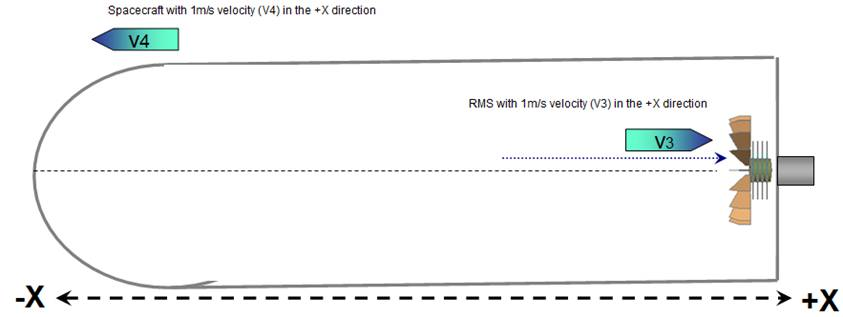Fig 7

Cycle 4

At this cycle the RMA is traveling (RMA’s rotors are off) with a 1 m/s velocity (V3) in the +X direction and the spacecraft with a 1 m/s velocity (V4) in the –X direction.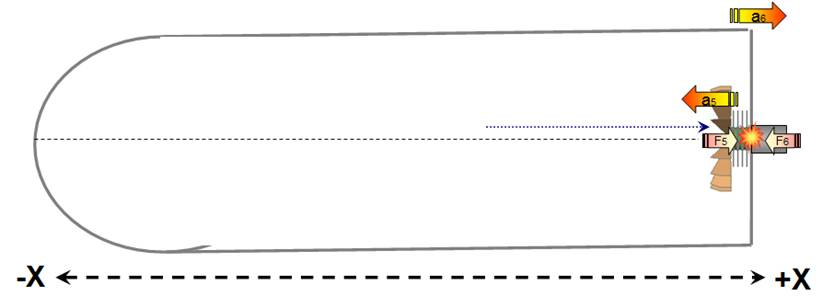Fig 8

Cycle 5

The RMA collides with the spacecraft’s inner wall with force F5 giving it a +X acceleration (a5).

Resulting changes in acceleration on system (spacecraft) in cycles 1 to 5

Cycle 1 produces acceleration (a2) in the +X direction.

Cycle 3 produces acceleration (a4) in the -X direction.

Cycle 5 produces acceleration (a5) in the +X direction.

Cycles 2 and 4 do not affect the spacecraft’s acceleration sufficiently to alter the final result.

For simplicity, we shall assume that in the presented example no final change in acceleration affects the system (spacecraft), this is possible if (a2) + (a5) = (a4)

We must not forget that acceleration a4 is a product of force F4, and force F4 can be increased or deceased by modifying the time interval the RMA’s rotors are blowing air molecules against the spacecrafts inner –X wall (can be also be modified by other factors.)

Now we will see what effect the activation of the RMA’s air brake in cycle 2 has on force F4.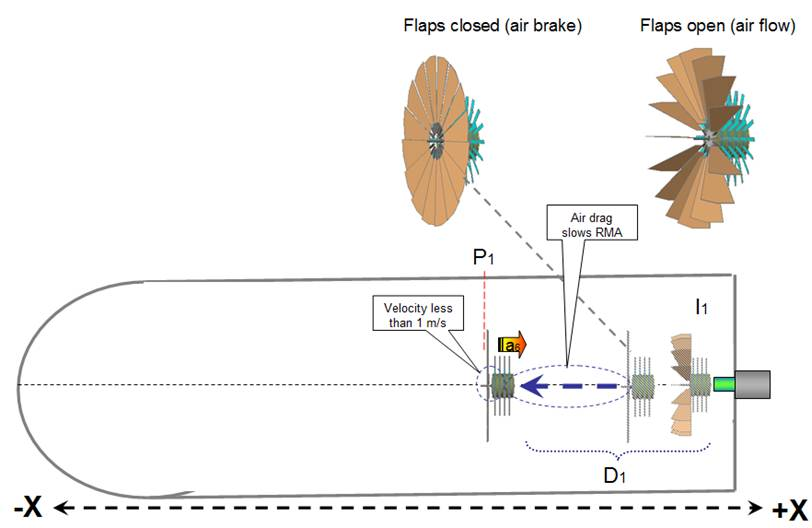Fig 9

Cycle 2.1 (Modified, with air brake activated)

The instant the RMA is pushed by the ramming piston 23 (cycle 1 fig 4) in the –X direction obtaining a velocity of 1m/s, the flaps turn (almost) 90 degrees to closed position offering maximum air drag during its travel of distance D1, because of the increased drag, the RMA’s relative velocity decreases as it travels distance D1 and will be traveling at LESS than 1m/s. when it reaches position P1.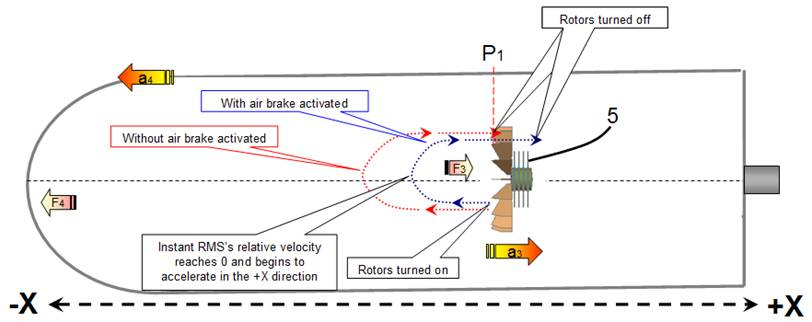Fig 10

Cycle 3.1 (Modified)

The instant the RMA reaches position P1, its flaps return to their original position (open) and the RMA rotor’s are activated blowing air in the –X direction, this creates a force (F3) that accelerate (a3) the RMA in the +X direction

As the RMA’s relative velocity was decreasing when it traveled distance D1, it arrives at point P1 with a velocity that is less than 1 m/s in the –X direction therefore it needs a smaller time interval to reach a relative velocity of 0, thus the rotors spend less time blowing air in the –X direction consequently the force F4 against the spacecraft’s –X inner wall is less and the spacecraft receives a lesser amount of acceleration in the –X direction.

As we have reduced the –X acceleration maintaining the +X acceleration unchanged the spacecraft will accelerate with each cycle for as long a time as electricity is transmitted to the RMA’s motor.

Experimental evidence

This page instructs how to set up a test bed to observe the effect.

The described experiment is designed as an inexpensive DIY setup so it can be duplicated with minimum time and expense.

Appendix A (Details of the cycles)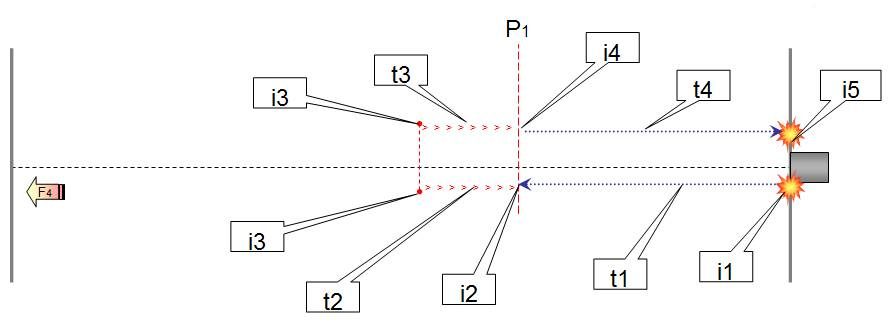Fig 11

Detail of the cycles without activating air brake (Fig 11)

 Description Force on spacecraft 1 Instant 1 (i1) Ramming rod gives the RMA a 100n push in the –X direction 100n in the +X direction (F2) 2 Time interval 1 (t1) RMA travels at a constant velocity of 1m/s in the –X direction 0 3 Instant 2 (i2) RMA arrives at position P1 with a 1m/s velocity, rotors are activated blowing air in the –X direction 4 Time interval 2 (t2) The breeze generated by the rotors collide with the spacecrafts –X inner hull incrementing force F4 (in the –X direction) every second the rotors are blowing air in the –X direction. The RMA’s slows its relative –X velocity. Force F4 (in the –X direction) is incremented every second the rotors are pushing air in the –X direction. 5 Instant 3 (i3) The RMA’s relative –X velocity reaches 0, and starts acceleration in the +X direction 6 Time interval 3 (t3) The RMA’s increases its relative +X velocity. Force F4 (in the –X direction) is incremented every second the rotors are pushing air in the –X direction. Note: Force F4 is directly proportional to the length of time intervals t2 and t3. 7 Instant 4 (i4) RMA obtains a relative +X velocity of 1m/s and rotors are deactivated. 8 Time interval 4 (t4) RMA travels at constant velocity of 1m/s in the +X direction 0 9 Instant 5 (i5) RMA traveling at 1m/s collides with the spacecrafts inner +X wall 100n in the +X direction (F5) (An electromagnet is activated to attaching the RMA to the spacecraft’s wall to prevent a “bounce effect”). We can conclude that if there is no acceleration gain by the spacecraft it is because the breeze generated during time intervals t2 and t3 is sufficient to create a force F4 that is exactly equal to the opposite forces (F2 and F5). We can also assume that in the described premise time intervals T1 and T4 are equal.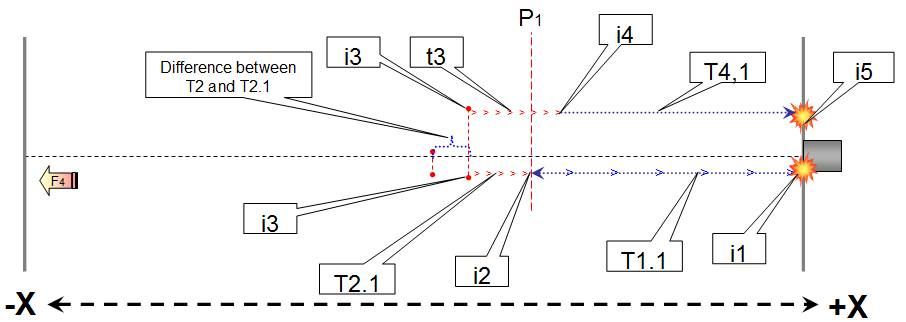Fig 12

Detail of the cycles activating the air brake (Fig 12)

 Description Force on spacecraft 1 Instant 1 (i1) Ramming rod gives the RMA a 100n push in the –X direction 100n in the +X direction (F2) 2 Time interval 1.1 (t1.1) RMA’s air brake is activated, the additional drag decreases the RMA’s –X velocity. The RMA takes more time to arrive at position P1 but change in time interval T1.1 has no effect on the spacecraft’s acceleration. 0 3 Instant 2 (i2) RMA arrives at position P1 with less than 1 m/s velocity, RMA’s air brake is deactivated, rotors are activated blowing air in the –X direction 4 Time interval 2.1 (t2.1) The RMA’s slows its relative –X velocity. (Needs less time to reach a relative velocity of 0 than time interval t2 because its starting velocity is less than 1 m/s. Therefore t2 > t2.1) The breeze generated by the rotors collide with the spacecrafts –X hull incrementing F4 (in the –X direction) every second the rotors are blowing air in the –X direction 5 Instant 3 (i3) The RMA’s relative –X velocity reaches 0, and starts acceleration in the +X direction 6 Time interval 3 (t3) The RMA’s increases its relative +X velocity. Force F4.1 (in the –X direction) is incremented every second the rotors are pushing air in the –X direction. Note: As t2 > t2.1 and forces F4 and F4.1 are directly proportional to the length of time intervals air is blown in the –X direction we have: F4 = t2 + t3 And F4.1 = t2.1 + t3 As t2 > t2.1 then F4 > F4.1 As the forces accelerating the spacecraft F2 and F5 are not affected by the use of the RMA’s air brake we can assume a net +X force is exerted on the spacecraft every cycle. Experiments where you can observe the propulsion effect. 7 Instant 4. (i4.) RMA obtains a relative +X velocity of 1m/s, rotors are deactivated. 0 8 Time interval 4.1 (t4.1) RMA travels at constant velocity of 1m/s in the +X direction 0 9 Instant 5 (i5) RMA traveling at 1m/s collides with the spacecrafts inner +X wall 100n in the +X direction (F5) (An electromagnet is activated to attaching the RMA to the spacecraft’s wall to prevent a “bounce effect”). We can conclude that as the –X force F4.1 is less than –X force F4 but +X forces F2 and F5 remain unchanged, the end result is a net +X force on the spacecraft.